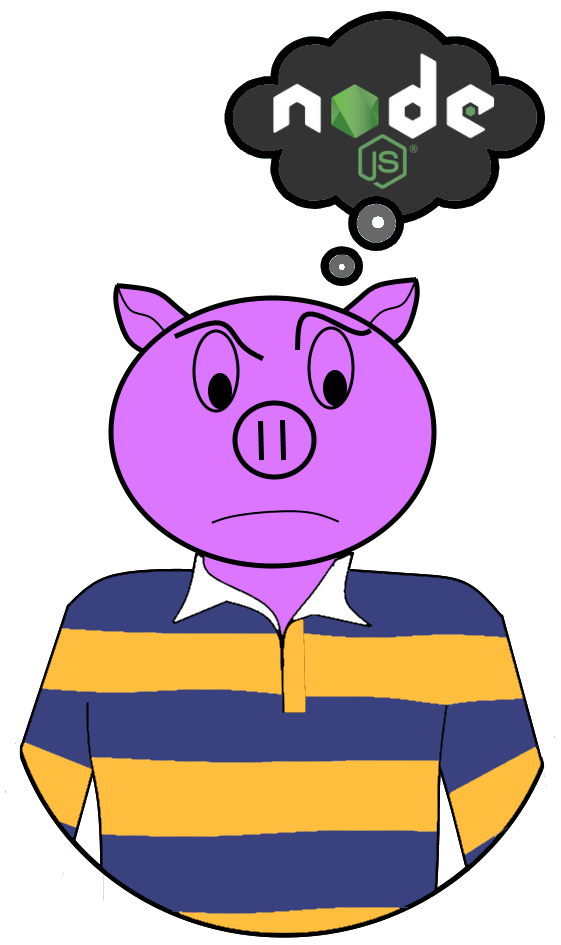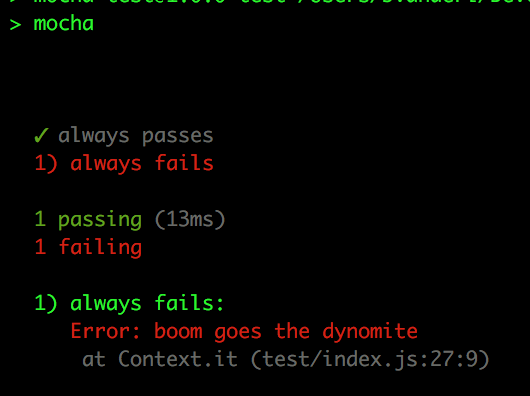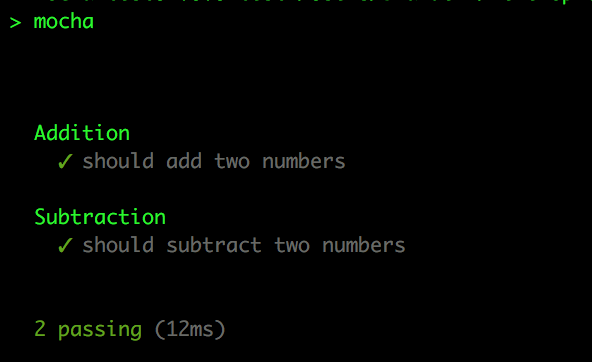# ImaginativeThinking.ca

A developers blog

# What the Heck is Mocha?

By:Brad

Hey Brad I’m starting to write tests for a Node application and I hear I’m suppose to use `mocha`, what the heck is that exactly?`mocha` is a testing framework for JavaScript/Node. It includes a command line tool for invoking tests and APIs for defining and setting up tests.

## Running Mocha Tests

To run tests with mocha you can use the command line tool `> mocha`.
This tool by defaults looks for tests defined under a sub folder called `test` but you can pass in a custom path to a directory or file `> mocha tests` or `> mocha test/mytest.js`. The command line has a number of arguments which you can find documented here.

In Node projects a NPM command is typically setup to call `mocha` with any and all arguments so you simply need to run `npm test` to invoke the mocha tests.

## How Mocha tests work

A mocha test is just plain old JavaScript, you simply need to register the test with `mocha` so it gets picked up and run by the command line tool.

To define a `mocha` test you simply need to use the `it()` function which comes with the `mocha` NPM module.

The `it()` function takes two parameters: a descriptive name which will be displayed in the terminal and a function to invoke.

```function doTest() {...}
it('my test', doTest);
```

The `doTest` function is responsible for invoking the code your testing and asserting that the code behaved as expected.

```it('should add two numbers', () => {
const result = 5 + 5;
chai.expect(result).to.equal(10);
});
```

Notice I wrote my description as a sentence which starts with it, isn’t that very descriptive: it should add two numbers

Above I’ve defined a test, I provided the test function as an inline arrow function for simplicity. In the test I call the code I’m testing, the addition operator, then used the `chai.expect` library to ensure that the addition operator added the two numbers together as expected.

If the test is successful `mocha` will report a good test, if the addition operator has a bug then `mocha` will report a failing test.

### But how does mocha know if the test passed or failed?

I see you are paying attention, if the assertion library isn’t part of `mocha` how does `mocha` know if the assertion failed?

Although you can just trust the magic that is `mocha` it can be handy to understand what `mocha` is actually doing when it runs the test. The arrow function I passed into the `it()` function is getting invoked by `mocha` when I run the test but it is getting wrapped in a try-catch under the hood. When invoked if no exception is thrown then the test passed, otherwise `mocha` catches the exception and marks the test failed.

```it('always passes', () => {
// No assertions means no exceptions
// means always passes
});

// Here an exception is thrown so mocha is catching it and
// marking the test failed. It will output the error text
// in the terminal
it('always fails', () => {
throw new Error('boom goes the dynamite');
});
```Exception? What you talking about? I’m not throwing an Exception!

The `chai.expect()` function I’m using here to assert the code’s behaviour will validate that the `result` equals what I expect it to (`10`) and if it does not match my expectation it will throw an exception.

The above test could be rewritten without `chai.expect` as so:

```it('should add two numbers', () => {
const result = 5 + 5;

if (result !== 10) {
throw new Error(`expect 10 got \${result}`);
}
});
```

As you can tell having an assertion library is pretty handy to keep the test LOC count down.

## Organizing Tests

Now that you know how to write a test lets talk about how to keep your tests organized.

You can just write a flat file with nothing but `it()` functions where each `it()` function defines another test case but `mocha` has another API used to define a Test Suite.

A TestSuite is a collection of tests related to testing different cases of a given component.

Lets say I have a bunch of tests for testing the addition operator and now I want to add tests for the subtraction operator. I could just keep writing `it()` functions but that might make it hard to sift through the tests when I run them or when I’m adding or modifying tests.

Luckily `mocha` lets you define TestSuites via the `describe()` function.

Just like the `it()` function `describe()` takes two parameters: a description of the suite and a function to call.

```describe('Addition', () => {
it('should add two numbers', () => {
const result = 5 + 5;
chai.expect(result).to.equal(10);
});
});

describe('Subtraction', () => {
it('should subtract two numbers', () => {
const result = 5 - 5;
chai.expect(result).to.equal(0);
});
});
```

For the most part the `describe()` function will be used to organize tests. I can, if my editor supports it, minimize all the addition tests and only look at the subtraction tests I can even tell `mocha` to only run the subtraction tests by adding `.only` to the `describe()` function.

```describe('Addition', () => {
it('should add two numbers', () => {
const result = 5 + 5;
chai.expect(result).to.equal(10);
});
});

// will only run the Subtraction tests
describe.only('Subtraction', () => {
it('should subtract two numbers', () => {
const result = 5 - 5;
chai.expect(result).to.equal(0);
});
});
```

The terminal output will also organize the test by adding the test suite description to each test. Depending on the reporter used they might even be indented.The secondary benefit that `describe()` blocks have is that the can set scope to your tests.

```describe('Addition', () => {
const expect = 10;
it('should add two numbers', () => {
const result = 5 + 5;
chai.expect(result).to.equal(expect);
});
});

describe('Subtraction', () => {
it('should subtract two numbers', () => {
const result = 5 - 5;
chai.expect(result).to.equal(0);
});
});
```

Here within my Addition `describe()` I defined a variable to be used in my `it()` tests. Thanks to the addition of block level scope in JavaScript with `const`/`let` this variable is only accessible to my Addition tests.

A better example as to why scoped variables within `describe()` blocks can be handy would be if you were testing a function which return a user object. The user object might contain multiple properties; instead of defining it over and over again you can define one globally for all get user tests.

```const { expect } = chai;
describe('Get User', () => {
const user = {
id: 12345,
name: 'Roger',
email: 'roger.wilco@sierra.com',
};

it('should return the user', () => {
expect(getUser(12345)).to.deep.equals(user);
});

it('should return all users', () => {
expect(getUsers()).to.deep.equals([user]);
});
});
```

## Setting up and Tearing down Tests

One other feature `mocha` has it the ability to run code before and after invoking tests.

### Setting Up

For example lets say we needed to seed a database with data prior to running tests. We can do that via the `before()` function. Like `describe()` and `it()` the `before()` function takes two parameters: a description, used if the before function failed, and a function to invoke.

```const { expect } = chai;
describe('Get User', () => {
const user = {
id: 12345,
name: 'Roger',
email: 'roger.wilco@sierra.com',
};

before('seed database', () => {
db.write(user);
});

it('should return the user', () => {
expect(getUser(12345)).to.deep.equals(user);
});

it('should return all users', () => {
expect(getUsers()).to.deep.equals([user]);
});
});
```

Here the `before()` function will be run just before invoking the two `it()` tests. The `before()` function will only be run once. If we need it to run before each `it()` test, so twice here, we could use the `beforeEach()` function instead. `beforeEach()` and `before()` work exactly the same way say for the frequency of there invocation.

Like the `const` variables `before()`/`beforeEach()` functions are scoped to the describe block:

```const { expect } = chai;
describe('Get User', () => {
...
before('seed database', () => {
db.write(user);
});
...
});

describe('Delete User', () => {
...
before('seed database', () => {
db.write(user);
});
...
});
```

Here `mocha` will first run the Get User suite where it will invoke its `before()` function prior to running the tests. Next `mocha` will run the Delete User suite where it will run its `before()` function prior to running that suites tests.

A suite can also have multiple `before()` blocks defined:

```const { expect } = chai;
describe('Get User', () => {
...
before('connect database', () => {
db.connect(connectionString);
});

before('seed database', () => {
db.write(user);
});
...
});
```

The order `before()` functions are called is the same as the order they appear in the `describe()` function. Here we would first connect to the database followed by seeding it. Reasons to define your setup steps in multiple `before()` functions could be for code clarity or for easier trouble shooting because the `before()` description will be displayed in the terminal if a `before()` function failed. If we had a single `before()` function the terminal might read before hook failed: connect and seed database where as by having two `before()` functions as we do here the terminal might read before hook failed: seed database allowing us to know we connected but failed to seed.

It does not matter where you put the `before()` functions within your suite but I’d recommend putting them first:

```const { expect } = chai;
describe('Get User', () => {
it('my test', () => {...});
...
before('seed database', () => { // still runs before the it()
db.write(user);
});
...
});
```

### Tearing Down

As eluded to earlier `mocha` will let you run code after a test run via the `after()` function. Similar to the `before()` function the `after()` function takes two parameters: A description and a function to call.

The `after()` function is run after all the tests of a given suite have been run. You can use the `after()` function to tear down your test setup.

```const { expect } = chai;
describe('Get User', () => {
...
before('connect database', () => {
db.connect(connectionString);
});

before('seed database', () => {
db.write(user);
});

after('flush database', () => {
db.destroy(user);
});
...
});
```

You can have multiple `after()` functions like you can the `before()` functions where the order of execution is the order they appear in the scope. There is also an `afterEach()` version which runs after each `it()` function.

It does not matter where you put the `after()`/`afterEach()` function in your suite, they will always be run after the tests. I’d just recommend putting them after the `before()` functions and before the `it()` functions to keep them close to their `before()` counter parts but if you put them after the `it()` functions I guess that is ok too.

```const { expect } = chai;
describe('Get User', () => {
after('flush database', () => { // still run after the it()
db.destroy(user);
});
...
before('seed database', () => {
db.write(user);
});

after('flush database', () => { // still run after the it();
db.destroy(user);
});

it('my tests', () => {...});

after('flush database', () => { // still run after the it();
db.destroy(user);
});
...
});
```

There you have it, that is what `mocha` is; a testing framework used to write tests for JavaScript/Node applications. It is installed via an NPM module which includes APIs for defining tests (`describe()`, `before()`/`beforeEach()`, `after()`/`afterEach()`, `it()`) and a command line tool for running the tests.

Until next time think imaginatively and design creatively